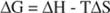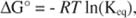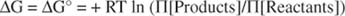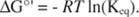## Energy Flow

Thermodynamics is the branch of chemistry and physics that deals with the energy flow in physical systems. The First Law of Thermodynamics states that energy in a system is neither created nor destroyed. The Second Law of Thermodynamics deals with the question of whether a reaction will occur: Spontaneous reactions occur with an increase in the entropy of a system; that is, the overall disorder of the system will increase. Entropy can be thought of as the energy that is not available to do work. For example, the oceans contain vast amounts of thermal energy in the form of the motions of individual water molecules. Yet this energy cannot be extracted to power a boat—that requires fuel or wind energy.

The amount of energy available for work is termed the free energy of a system and is defined as the difference in heat content between the products and reactants, less the amount of entropy change (multiplied by the temperature of the system):where ΔG is the amount of free energy released from the reaction, ΔH is the change in heat content, or enthalpy, T is the temperature in degrees Kelvin, and ΔS is the change in entropy. Another way of stating the Second Law of Thermodynamics is that reactions occur in the direction in which the free energy change is negative.

The change in free energy of a reaction in the standard state (conventionally, all reactants and products at 1M) is related to the equilibrium constant for the reaction by the following relation:where R is the gas constant, T is the temperature in degrees Kelvin, and K eq is the equilibrium constant for the reaction. A reaction that is favored has K eq > 1, and a reaction that is unfavored has K eq<1. In the former case, ln(K eq) is positive, so the free energy change of a favored reaction is negative; it is exergonic . Conversely, the natural logarithm of a number less than 1 is negative; because the product of two negative numbers is positive, the free energy change of an unfavored reaction is positive; it is endergonic .

Free energy changes associated with a biochemical reaction are determined at a standard state, with all reactants and products at 1M. Many biomolecules are unstable in acid, so the biochemical standard state is set at pH = 7.0 rather than at pH = 0 (1M acid), the standard state for chemical reactions. Biochemical standard free energies of reaction are given as Δ ’ to where the ‘indicates this change in standard conditions.

Biomolecules are also present in much lower concentrations than the standard state of 1M. The free energy change associated with reactions under conditions other than the standard state is given by the relationship:where Π [Products] represents the concentrations of the products of the reaction, multiplied together, and Π [Reactants] represents the concentrations of the reactants, multiplied together. (If more than one molecule of a product or reactant is involved in a reaction, the term for that component is raised to the power of the number of molecules involved in the reaction, just as for any chemical reaction.) R is the gas constant, and T is the absolute temperature of the reaction. This relationship reduces to ΔG = ΔG° = when all the products and reactants are present at 1M concentration, the standard state. At equilibrium, ΔG = 0, and the equation reduces to:K eq is simply the equilibrium constant of the reaction at pH 7.0.

Back to Top
A18ACD436D5A3997E3DA2573E3FD792A